# Using AI to Super Compress Images

Neural Network algorithms are showing promising results for different complex problems. Here we discuss how these algorithms are used in image compression.comments

Data driven algorithms like neural networks have taken the world by storm. They recent surge is due to several factors, including cheap and powerful hardware, and vast amounts of data. Neural Networks are currently the state of the art when it comes to ‘cognitive’ tasks like image recognition, natural language understanding , etc. ,but they don’t have to be limited to such tasks. In this post I will discuss a way to compress images using Neural Networks to achieve state of the art performance in image compression , at a considerably faster speed.

This article assumes some familiarity with neural networks, including convolutions and loss functions.

### What is image compression?

Image compression is the process of converting an image so that it occupies less space. Simply storing the images would take up a lot of space, so there are codecs, such as JPEG and PNG that aim to reduce the size of the original image.

#### Lossy vs. Lossless compression

There are two types of image compression: Lossless and Lossy.

As their names suggest, in Lossless compression, it is possible to get back all the data of the original image, while in Lossy, some of the data is lost during the conversion.

E.g. JPG is a lossy algorithm, while PNG is a lossless algorithm.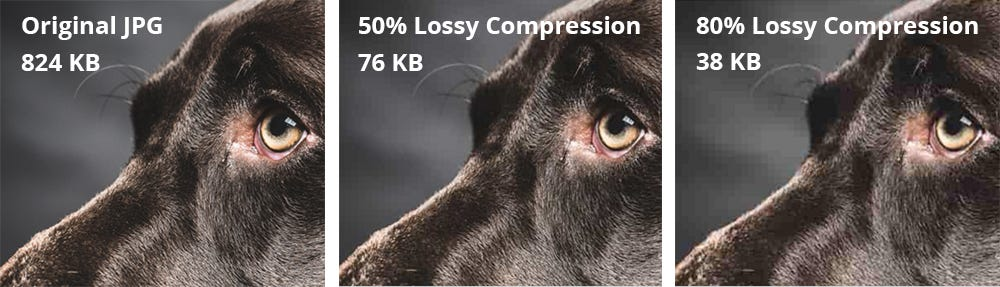Notice the image on the right has many blocky artifacts. This is how the information is getting lost. Nearby pixels of similar colors are compressed as one area, saving space, but also losing the information about the actual pixels. Of course, the actual algorithms that codecs like JPEG , PNG etc use are much more sophisticated, but this is a good intuitive example of lossy compression. Lossless is good, but it ends up taking a lot of space on disk.

There are better ways to compress images without losing much information, but they are quite slow, and many use iterative approaches, which means they cannot be run in parallel over multiple CPU cores, or GPUs. This renders them quite impractical in everyday usage.

### Enter Convolutional Neural Networks

If anything needs to be computed and it can be approximated, throw a neural network at it. The authors used a fairly standard Convolutional Neural Network to improve image compression. Their method not only performs at par with the ‘better ways’ (if not even better), it can also leverage parallel computing, resulting in a dramatic speed increase.

The reasoning behind it is that convolution neural networks(CNN) are very good at extracting spatial information from images, which are then represented in a more compact form (e.g. only the ‘important’ bits of an image are stored). The authors wanted to leverage this capability of CNNs to be able to better represent images.

### The Architecture

The authors proposed a dual network. The first network , which will take the image and generate a compact representation (ComCNN). The output of this network will then be processed by a standard codec (e.g. JPEG). After going through the codec, the image will be passed to a 2nd network, which will ‘fix’ the image from the codec, trying to get back the original image. The authors called it Reconstructive CNN (RecCNN). Both networks are iteratively trained, similar to a GAN.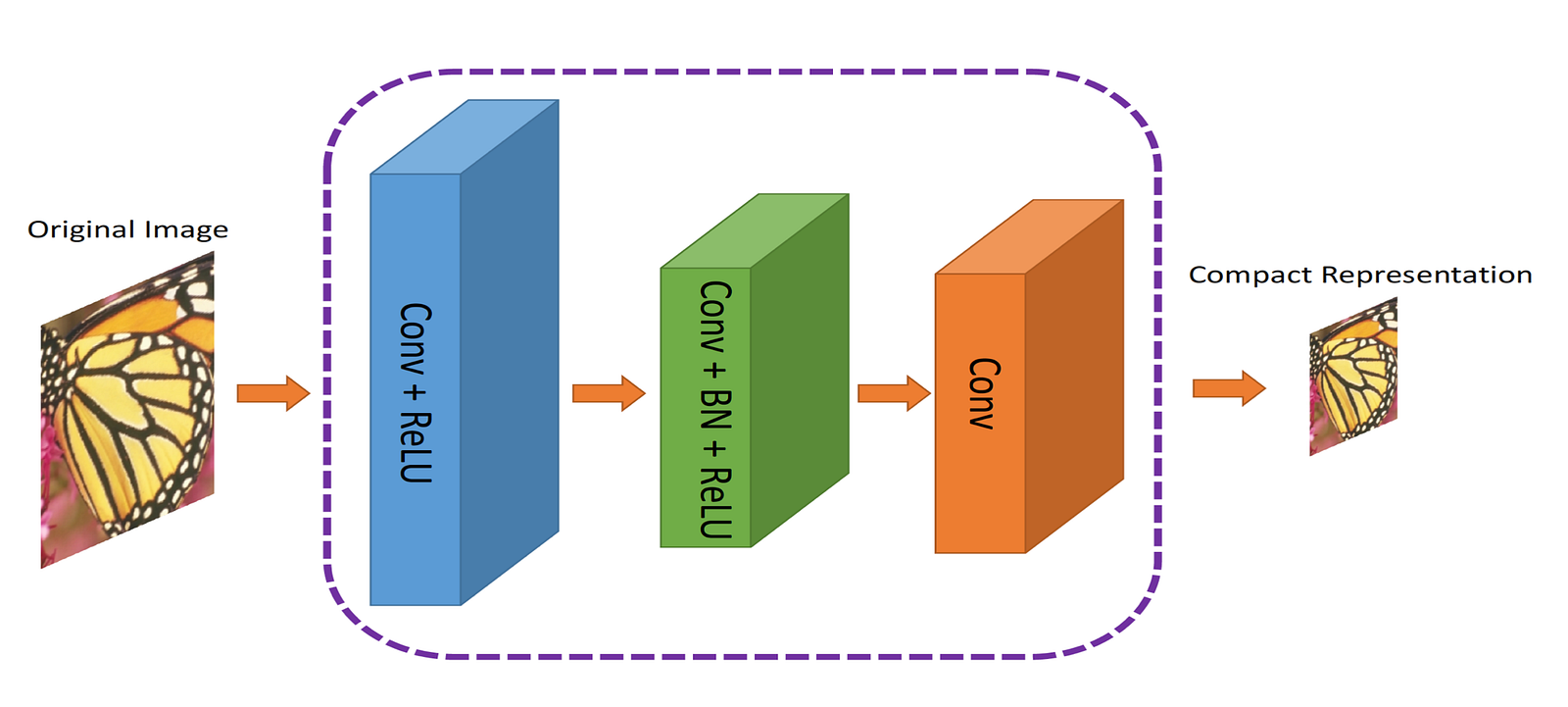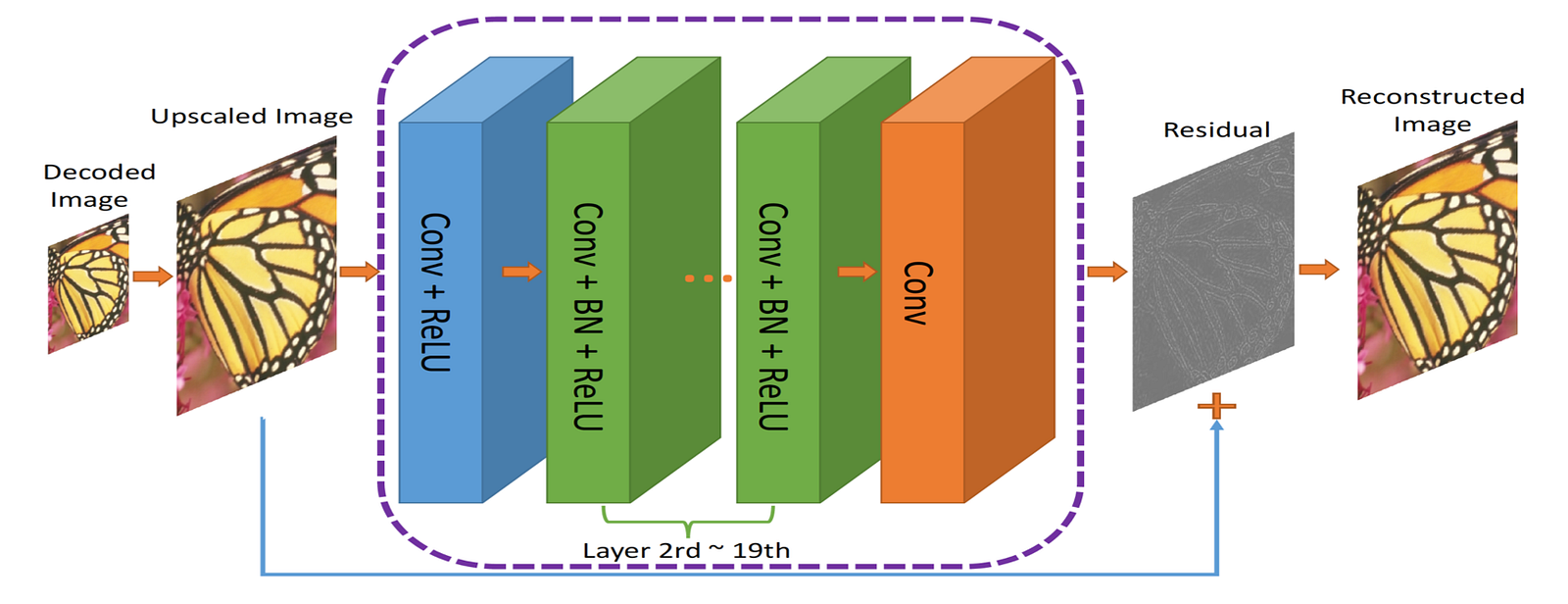The output from the codec is upscaled, then passed to RecCNN. The RecCNN will try to output an image that looks as similar to original image as possible.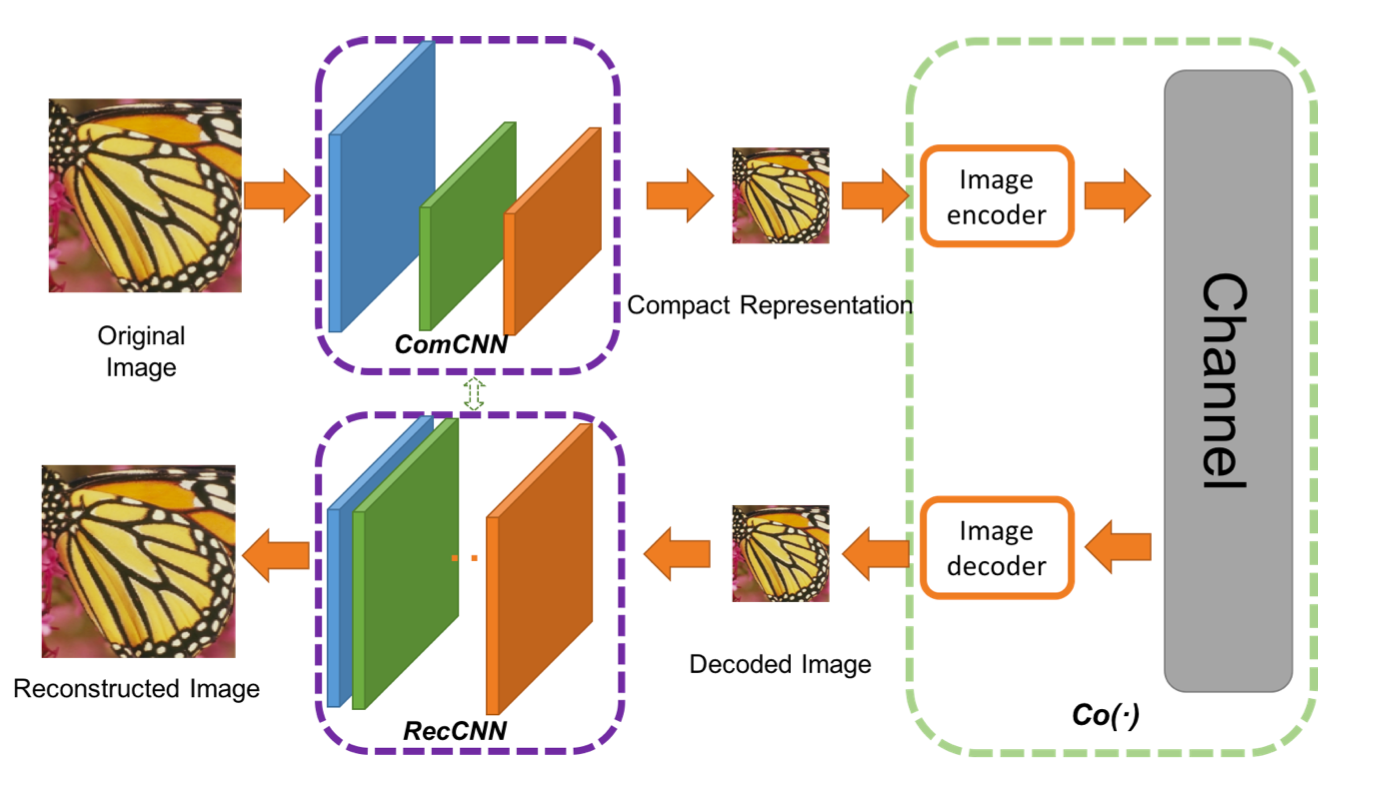### What is the residual?

The residual can be thought of as a post processing step to ‘improve’ the image that the codec decodes. The neural network, which has a lot of ‘information’ about the world, can make cognitive decisions about what to ‘fix’. This idea is based on residual learning , and you can read about it in depth here.

### The loss functions

Since there are two networks, two loss functions are used. The first one, for ComCNN, labeled as L1 is defined as: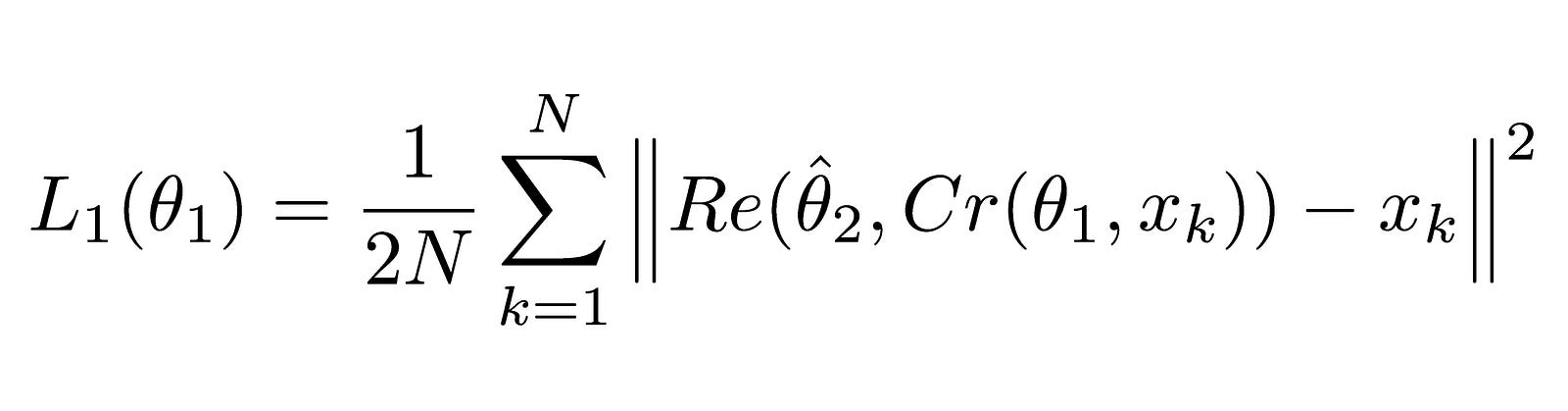#### Explanation

This equation may look complicated, but it is actually the standard (Mean Squared Error) MSE. The ||²s signify the ‘norm’ of the vector that they enclose.Equation 1.1

Cr denotes the output of the ComCNN. θ denotes the trainable parameters of ComCNN, and Xk denotes the input imageEquation 1.2

Re() denotes the RecCNN. This equation just passes the value of Equation 1.1 to the RecCNN. θ hat denotes the trainable parameters of RecCNN (the hat denotes that the parameters are fixed)

#### Intuitive definition

Equation 1.0 will make ComCNN modify its weights such that , after being recreated by RecCNN, the final image will look as close to the real input image as possible.

The second loss function , for RecCNN, is defined as: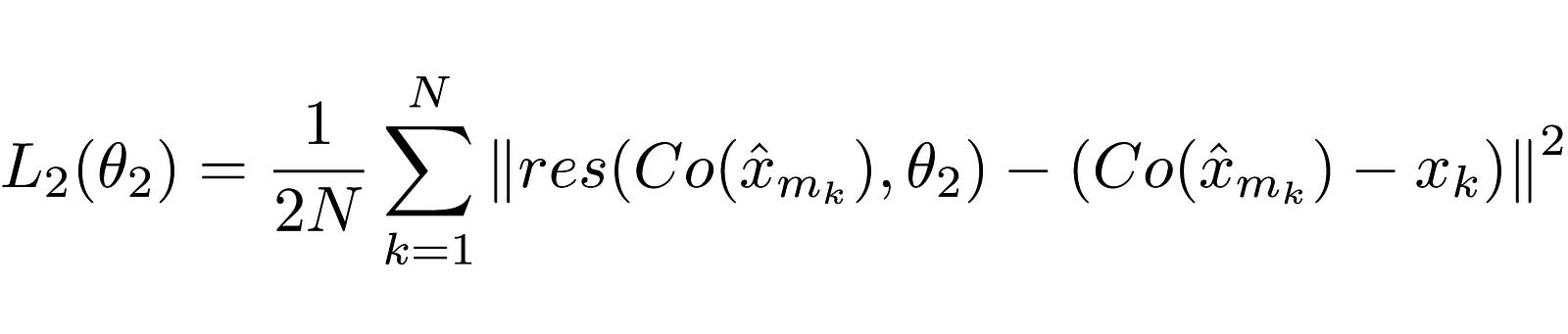#### Explanation

Again, the function may look complicated, but it is a mostly standard neural network loss function (MSE ).Co() denotes the output from the codec. x hat denotes the output from ComCNN. θ2 denotes the trainable parameters of RecCNN. res() just represents the residual that the network has learned. It is just the output of RecCNN. It is worth noting that RecCNN is trained on the difference between Co() and input image , and not directly from the input image.

#### Intuitive definition

Equation 2.0 will make RecCNN modify its weights such that the output of it looks as close to the original image as possible.

### The Training Scheme

The models are trained iteratively, similar to the way GANs are trained. One model’s weights are fixed while the other model’s weights are updated, then the other model’s weights are fixed, while the first model is trained.

### Benchmarks

The authors compared their method with existing methods, including plain codecs. Their method performs better than other methods, while maintaining high speeds when used on capable hardware. The authors tried using just one of the networks , and they noted that there were performance drops.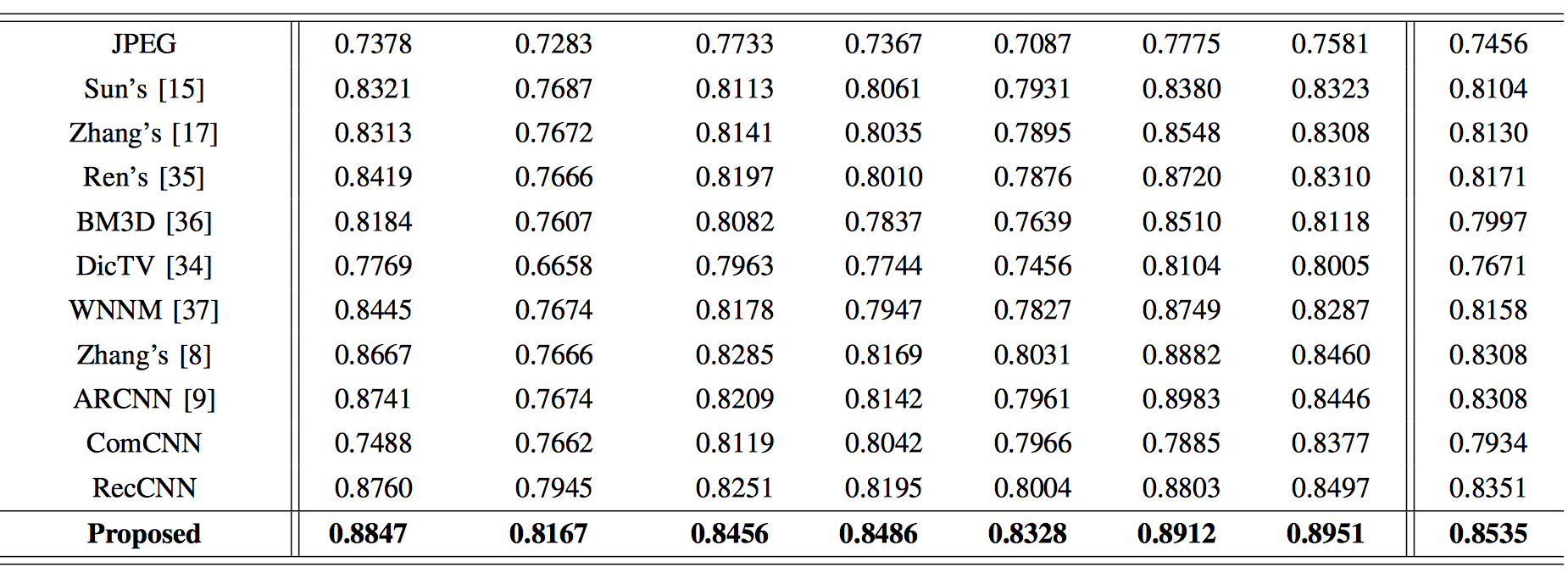### Conclusion

We took a look at a novel way of applying deep learning to compress images. We talked about the possibility of using neural networks on tasks besides ‘common’ ones such as image classification and language processing. This method is not only as good at the state of the art , but it can process images at a much faster rate.

Original. Reposted with permission.

Related: